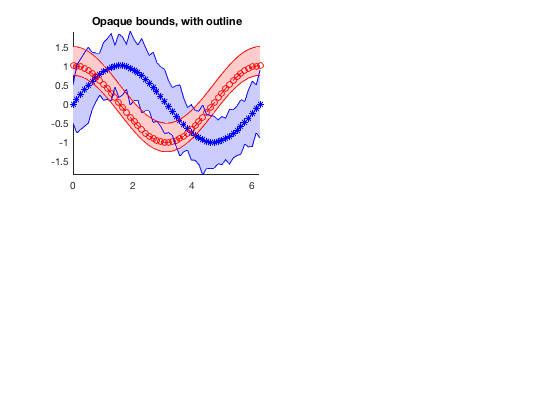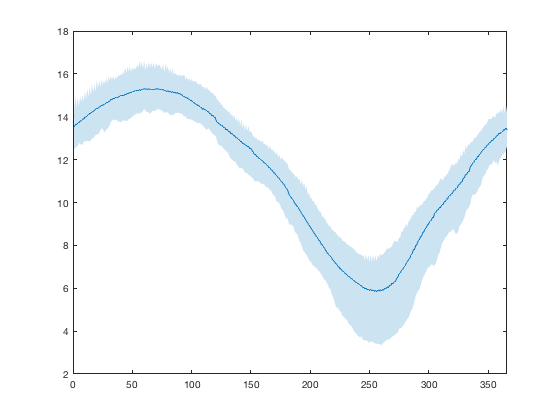# boundedline documentation

The boundedline function plots lines with shaded error/confidence bounds.

Back to Climate Data Tools Contents

## Syntax

```boundedline(x, y, b)
boundedline(x, y, b, linespec)
boundedline(x1, y1, b1, linespec1,  x2, y2, b2, linespec2)
boundedline(..., 'alpha')
boundedline(..., ax)
boundedline(..., 'transparency', trans)
boundedline(..., 'orientation', orient)
boundedline(..., 'nan', nanflag)
boundedline(..., 'cmap', cmap)
[hl, hp] = boundedline(...)```

## Description

boundedline(x, y, b) plots a line with coordinates given by x and y, surrounded by a patch extending a certain distance b above/below that line. The dimensions of the x, y, and b arrays can vary to allow for multiple lines to be plotted at once, and for patch bounds to be either constant or varying along the length of the line. The dimensions of x and y must meet the same requirements as for the plot function, and will produce the same number of lines as produced by plot with the same inputs. The b array should be a npoint x nside x nline array, with dimensions corresponding to each point along the line (dimension 1), each side of the line (lower/upper or left/right, depending on orientation) (dimension 2), and each plotted line described by the preceding x-y values (dimension 3). If size(b,1) == 1, the bounds will be the same for all points along the line. If size(b,2) == 1, the bounds will be symmetrical on both sides of the lines. If size(b,3) == 1, the same bounds will be applied to all lines described by the preceding x-y arrays (only applicable when either x or y is an array). Bounds cannot include Inf, -Inf, or NaN.

boundedline(..., 'alpha') renders the bounded area patch using a partially-transparent patch the same color as the corresponding line(s). If not included, the bounded area will use a fully-opaque patch in a lighter shade of the corresponding line color.

boundedline(..., 'transparency', transp) indicates the tranparency or intensity of the bounds patch, using a scalar between 0 and 1. Default is 0.2.

boundedline(..., 'orientation', orient) indicates the orientation of the bounds. Orientation can be either 'vert' for vertical (y-direction) bounds, or 'horiz' for horizontal (x-direction) bounds. Default is 'vert'.

boundedline(..., 'nan', nanflag) indicates how the bounds patch should handle NaNs in the line coordinates or bounds values. Options are 'fill', to smooth over the gap using neighboring values, 'gap' to leave a blank space in the patch at those points, or 'remove' to drop the NaN-points entirely, leading to linear interpolation of the gap in the patch. See examples below for more details on these options.

boundedline(..., 'color', col) specifies a line color.

boundedline(..., 'cmap', cmap) colors the lines (in order of plotting) acording to the colors in this n x 3 colormap array, overriding any linespec or default colors.

boundedline(..., ax) plots the bounded line to the axis indicated by handle ax. If not included, the current axis is used.

[hl, hp] = boundedline(...) returns the handles the resulting line and patch object(s) to hl and hp, respectively.

## Example 1: Plotting lines using various syntax options

This example builds the 4-panel example image used on the MatlabCentral File Exchange, which shows several different methods for supplying line coordinates, bounds coordinates, and shading options.

The first axis plots two lines using the LineSpec option for input, which allows yoy to set line color, line color, and marker type for each line. The bounds on the first line vary over x, while the bounds on the second line are constant for all x. An outline is added to the bounds so the overlapping region can be seen more clearly.

```x = linspace(0, 2*pi, 50);
y1 = sin(x);
y2 = cos(x);
e1 = rand(size(y1))*.5+.5;
e2 = [.25 .5];

ax(1) = subplot(2,2,1);
[l,p] = boundedline(x, y1, e1, '-b*', x, y2, e2, '--ro');
outlinebounds(l,p);
title('Opaque bounds, with outline');
axis tight;
```For our second axis, we use the same 2 lines, and this time assign x-varying bounds to both lines. Rather than using the LineSpec syntax, this example uses the default color order to assign the colors of the lines and patches. I also turn on the 'alpha' option, which renders the patch wit partial transparency.

```ax(2) = subplot(2,2,2);
boundedline(x, [y1;y2], rand(length(y1),2,2)*.5+.5, 'alpha');
title('Transparent bounds');
axis tight;
```The bounds can also be assigned to a horizontal orientation, for a case where the x-axis represents the dependent variable. In this case, the scalar error bound value applies to both lines and both sides of the lines.

```ax(3) = subplot(2,2,3);
boundedline([y1;y2], x, e1(1), 'orientation', 'horiz')
title('Horizontal bounds');
axis tight;
```Rather than use a LineSpec or the default color order, a colormap array can be used to assign colors. In this case, increasingly-narrower bounds are added on top of the same line.

```ax(4) = subplot(2,2,4);
boundedline(x, repmat(y1, 4,1), permute(0.5:-0.1:0.2, [3 1 2]), ...
'cmap', cool(4), ...
'transparency', 0.5);
title('Multiple bounds using colormap');

set(ax([1 2 4]), 'xlim', [0 2*pi]);
set(ax(3), 'ylim', [0 2*pi]);
axis tight;
```## Example 2: Common climate data uses

A typical use of this type of plot would be to show a range in values associated with a timeseries. For example, let's looks at the seasonal variation in sea ice extent data:

```S = load('seaice_extent.mat');
figure;
plot(doy(S.t), S.extent_N, '.');
set(gca, 'xlim', [0 366]);
```We can calculate the daily range across all years, and plot these bounds along with the mean

```[g, dy] = findgroups(doy(S.t));
icemin = splitapply(@min, S.extent_N, g);
icemax = splitapply(@max, S.extent_N, g);
icemean = splitapply(@mean, S.extent_N, g);
bnd = [icemean - icemin, icemax - icemean];

cla;
boundedline(dy, icemean, bnd);
```One thing to note when plotting timeseries is that patch objects do not currently support datetime input, and therefore neither can boundedline at this time; you must first convert datetime input data to datenumbers to plot with this function, and cannot target a datetime axis.

## Example 3: Filling gaps

If you plot a line with one or more NaNs in either the x or y vector, the NaN location is rendered as a missing marker with a gap in the line. However, the patch command does not handle NaNs gracefully; it simply fails to show the patch at all if any of the coordinates include NaNs.

Because of this, the expected behavior of the patch part of boundedline when confronted with a NaN in either the bounds array (b) or the x/y-coordinates of the line (which are used to calculate the patch coordinates) is ambiguous. I offer a few options.

Before I demonstrate the options, I'll create a dataset that has a few different types of gaps:

```x = linspace(0, 2*pi, 50);
y = sin(x);
b = [ones(size(y))*0.2; rand(size(y))*.5+.5]';

y(10)   = NaN;  % NaN in the line but not bounds
b(20,1) = NaN;  % NaN in lower bound but not line
b(30,2) = NaN;  % NaN in upper bound but not line
b(40,:) = NaN;  % NaN in both sides of bound but not line
```

Here's what that looks like in an errorbar plot.

```figure;
he = errorbar(x,y,b(:,1), b(:,2), '-bo');

line([x([10 20 30 40]); x([10 20 30 40])], [ones(1,4)*-2;ones(1,4)*2], ...
'color', ones(1,3)*0.5, 'linestyle', ':');
text(x(10), sin(x(10))-0.2, {'\uparrow','Line','gap'}, 'vert', 'top', 'horiz', 'center');
text(x(20), sin(x(20))-0.2, {'\uparrow','Lower','bound','gap'}, 'vert', 'top', 'horiz', 'center');
text(x(30), sin(x(30))-0.2, {'\uparrow','Upper','bound','gap'}, 'vert', 'top', 'horiz', 'center');
text(x(40), sin(x(40))-0.2, {'\uparrow','Two-sided','bound','gap'}, 'vert', 'top', 'horiz', 'center');

axis tight equal;
```The default method for dealing with NaNs in boundedline is to leave the gap in the line, but smooth over the gap in the bounds based on the neighboring points. This option can be nice if you only have one or two missing points, and you're not interested in emphasizing those gaps in your plot:

```delete(he);
[hl,hp] = boundedline(x,y,b,'-bo', 'nan', 'fill');
ho = outlinebounds(hl,hp);
set(ho, 'linestyle', ':', 'color', 'r', 'marker', '.');
```I've added bounds outlines in a contrasting color so you can see how I'm handling individual points.

The second option leaves a full gap in the patch for any NaN. I considered allowing one-sided gaps, but couldn't think of a good way to distinguish a gap from a zero-valued bound. I'm open to suggestions if you have any (email me).

```delete([hl hp ho]);
[hl,hp] = boundedline(x,y,b,'-bo', 'nan', 'gap');
ho = outlinebounds(hl,hp);
set(ho, 'linestyle', ':', 'color', 'r', 'marker', '.');
```The final option removes points from the patch that are NaNs. The visual result is very similar to the fill option, but the missing points are apparent if you plot the bounds outlines.

```delete([hl hp ho]);
[hl,hp] = boundedline(x,y,b,'-bo', 'nan', 'remove');
ho = outlinebounds(hl,hp);
set(ho, 'linestyle', ':', 'color', 'r', 'marker', '.');
```## Author Info

This function and supporting documentation was written by Kelly Kearney for the Climate Data Toolbox for Matlab, 2019. It is available as part of this toolbox, and can also be downloaded individually from GitHub.